Home
Hostname: page-component-cf9d5c678-xvx2z Total loading time: 0.197 Render date: 2021-08-03T09:52:08.813Z Has data issue: true Feature Flags: { "shouldUseShareProductTool": true, "shouldUseHypothesis": true, "isUnsiloEnabled": true, "metricsAbstractViews": false, "figures": true, "newCiteModal": false, "newCitedByModal": true, "newEcommerce": true, "newUsageEvents": true }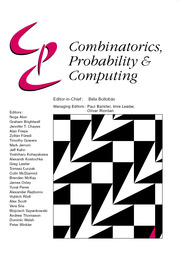Combinatorics, Probability and Computing

# Sharp bounds for decomposing graphs into edges and triangles

Published online by Cambridge University Press:  12 October 2020

Corresponding

## Abstract

For a real constant α, let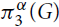$\pi _3^\alpha (G)$ be the minimum of twice the number of K2’s plus α times the number of K3’s over all edge decompositions of G into copies of K2 and K3, where Kr denotes the complete graph on r vertices. Let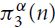$\pi _3^\alpha (n)$ be the maximum of$\pi _3^\alpha (G)$ over all graphs G with n vertices.

The extremal function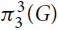$\pi _3^3(n)$ was first studied by Győri and Tuza (Studia Sci. Math. Hungar. 22 (1987) 315–320). In recent progress on this problem, Král’, Lidický, Martins and Pehova (Combin. Probab. Comput. 28 (2019) 465–472) proved via flag algebras that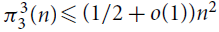$\pi _3^3(n) \le (1/2 + o(1)){n^2}$. We extend their result by determining the exact value of$\pi _3^\alpha (n)$ and the set of extremal graphs for all α and sufficiently large n. In particular, we show for α = 3 that Kn and the complete bipartite graph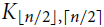${K_{\lfloor n/2 \rfloor,\lceil n/2 \rceil }}$ are the only possible extremal examples for large n.

## MSC classification

Type
Paper
Information
Combinatorics, Probability and Computing , March 2021 , pp. 271 - 287
Creative CommonsThis is an Open Access article, distributed under the terms of the Creative Commons Attribution licence (http://creativecommons.org/licenses/by/4.0/), which permits unrestricted re-use, distribution, and reproduction in any medium, provided the original work is properly cited.

## Footnotes

Research supported in part by NSF grants DMS-1600390 and DMS-1855653.

Supported by the European Research Council (ERC) under the European Union’s Horizon 2020 research and innovation programme (grant agreement 648509). This publication reflects only its authors’ view; the European Research Council Executive Agency is not responsible for any use that may be made of the information it contains.

§

Research supported in part by NSF grants DMS-1600483 and DMS-1855622.

Supported by ERC grant 306493 and EPSRC grant EP/K012045/1 and by Leverhulme research project grant RPG-2018-424.

ǁ

Previous affiliation: Faculty of Informatics, Masaryk University, Botanická 68A, 602 00 Brno, Czech Republic. This project has received funding from the European Union’s Horizon 2020 research and innovation programme under the Marie Skłodowska-Curie grant agreement 800607. This publication reflects only its authors’ view; the European Research Council Executive Agency is not responsible for any use that may be made of the information it contains.

## References

Alon, N.,Fischer, E.,Krivelevich, M. andSzegedy, M. (2000) Efficient testing of large graphs. Combinatorica 20 451–476.CrossRefGoogle Scholar
Barber, B.,Kühn, D.,Lo, A. andOsthus, D. (2016) Edge-decompositions of graphs with high minimum degree. Adv. Math. 288 337–385.Google Scholar
Bollobás, B. (1998) Modern Graph Theory. Springer.CrossRefGoogle Scholar
Chung, F. R. K. (1981) On the decomposition of graphs. SIAM J. Algebraic Discrete Methods 2 1–12.CrossRefGoogle Scholar
Delcourt, M. andPostle, L. (2019) Progress towards Nash-Williams’ conjecture on triangle decompositions. arXiv:1909.00514Google Scholar
Dross, F. (2016) Fractional triangle decompositions in graphs with large minimum degree. SIAM J. Discrete Math. 30 36–42.Google Scholar
Dukes, P. andHorsley, D. (2020) On the minimum degree required for a triangle decomposition. SIAM J. Discrete Math. 34 597–610.Google Scholar
Erdős, P. (1967) Some recent results on extremal problems in graph theory: results. In Theory of Graphs (Internat. Sympos., Rome, 1966), pp. 117–123 (English), pp. 124–130 (French). Gordon & Breach.Google Scholar
Erdős, P. (1971) Some unsolved problems in graph theory and combinatorial analysis. In Combinatorial Mathematics and its Applications (Proc. Conf., Oxford, 1969), pp. 97–109. Academic Press.Google Scholar
Erdős, P.,Goodman, A.W. and Pósa, L. (1966) The representation of a graph by set intersections. Canad. J. Math. 18 106–112.Google Scholar
Győri, E. (1988) On the number of edge-disjoint triangles in graphs of given size. In Combinatorics (Eger, 1987), Vol. 52 of Colloquia Mathematica Societatis János Bolyai, pp. 267–276. North-Holland.Google Scholar
Győri, E. (1991) On the number of edge disjoint cliques in graphs of given size. Combinatorica 11 231–243.CrossRefGoogle Scholar
Győri, E. (1992) Edge disjoint cliques in graphs. In Sets, Graphs and Numbers (Budapest, 1991), Vol. 60 of Colloquia Mathematica Societatis János Bolyai, pp. 357–363. North-Holland.Google Scholar
Győri, E. andKeszegh, B. (2017) On the number of edge-disjoint triangles in K 4-free graphs. Combinatorica 37 1113–1124.CrossRefGoogle Scholar
Győri, E. andKostochka, A. V. (1979) On a problem of G. O. H. Katona and T. Tarján. Acta Math. Acad. Sci. Hungar. 34 321–327.Google Scholar
Győri, E. andTuza, Z. (1987) Decompositions of graphs into complete subgraphs of given order. Studia Sci. Math. Hungar. 22 315–320.Google Scholar
Haxell, P.E. and Rödl, V. (2001) Integer and fractional packings in dense graphs. Combinatorica 21 13–38.CrossRefGoogle Scholar
Kahn, J. (1981) Proof of a conjecture of Katona and Tarján. Period. Math. Hungar. 12 81–82.Google Scholar
Král’, D.,Lidický, B.,Martins, T.L. and Pehova, Y. (2019) Decomposing graphs into edges and triangles. Combin. Probab. Comput. 28 465–472.Google Scholar
Liu, H.,Pikhurko, O. andStaden, K. (2020) The exact minimum number of triangles in graphs of given order and size. Forum Math. Pi 8 e8.CrossRefGoogle Scholar
Nash-Williams, C. (1970) An unsolved problem concerning decomposition of graphs into triangles. In Combinatorial Theory and Its Applications III, pp. 1179–1183. North-Holland.Google Scholar
Pikhurko, O. andRazborov, A. (2017) Asymptotic structure of graphs with the minimum number of triangles. Combin. Probab. Comput. 26 138–160.Google Scholar
Pyber, L. (1992) Covering the edges of a graph by …. In Sets, Graphs and Numbers (Budapest, 1991), Vol. 60 of Colloquia Mathematica Societatis János Bolyai, pp. 583–610. North-Holland.Google Scholar
Razborov, A. (2007) Flag algebras. J. Symb. Logic 72 1239–1282.Google Scholar
Razborov, A. (2008) On the minimal density of triangles in graphs. Combin. Probab. Comput. 17 603–618.Google Scholar
Simonovits, M. (1968) A method for solving extremal problems in graph theory, stability problems. In Theory of Graphs (Proc. Colloq., Tihany, 1966), pp. 279–319. Academic Press.Google Scholar
Tuza, Z. (2001) Unsolved combinatorial problems, Part I. BRICS Lecture Series LS-01-1.Google Scholar
Yuster, R. (2005) Integer and fractional packing of families of graphs. Random Struct. Algorithms 26 110–118.Google ScholarYou have AccessOpen access

# Send article to Kindle

Note you can select to send to either the @free.kindle.com or @kindle.com variations. ‘@free.kindle.com’ emails are free but can only be sent to your device when it is connected to wi-fi. ‘@kindle.com’ emails can be delivered even when you are not connected to wi-fi, but note that service fees apply.

Find out more about the Kindle Personal Document Service.

Sharp bounds for decomposing graphs into edges and triangles
Available formats
×

# Send article to Dropbox

To send this article to your Dropbox account, please select one or more formats and confirm that you agree to abide by our usage policies. If this is the first time you use this feature, you will be asked to authorise Cambridge Core to connect with your <service> account. Find out more about sending content to Dropbox.

Sharp bounds for decomposing graphs into edges and triangles
Available formats
×

# Send article to Google Drive

To send this article to your Google Drive account, please select one or more formats and confirm that you agree to abide by our usage policies. If this is the first time you use this feature, you will be asked to authorise Cambridge Core to connect with your <service> account. Find out more about sending content to Google Drive.

Sharp bounds for decomposing graphs into edges and triangles
Available formats
×
×